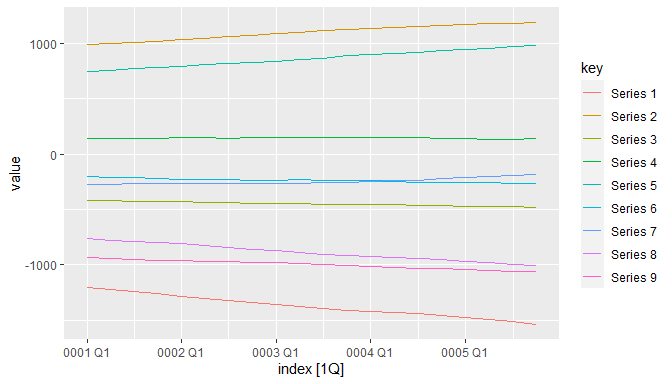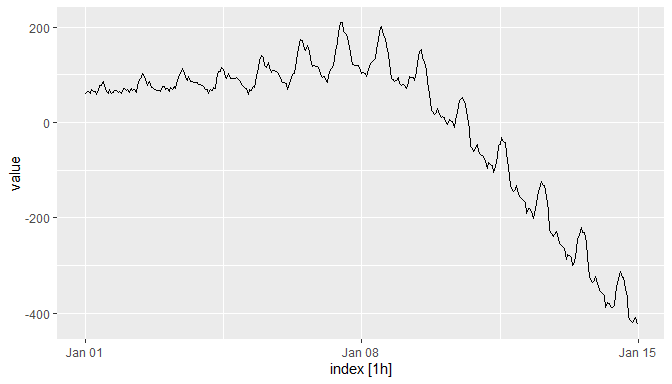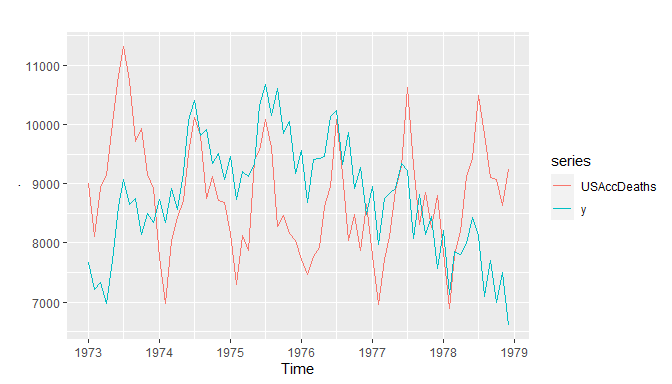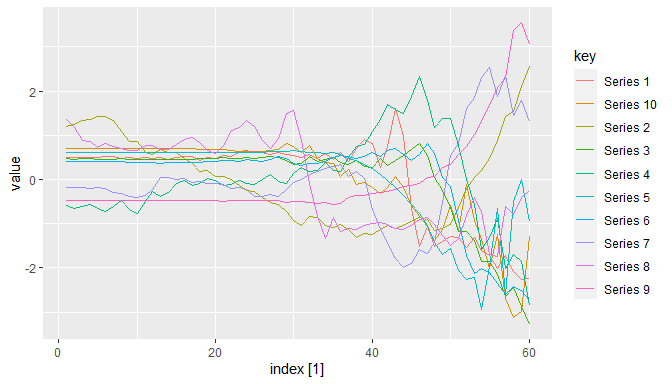# Introduction to gratis

## Warning: package 'knitr' was built under R version 4.0.5

The gratis package generates synthetic time series data with diverse and controllable characteristics. It uses Gaussian mixture autoregressive (MAR) models to generate a wide range of non-Gaussian and nonlinear time series. The theory and methods are described in Kang, Li and Hyndman (2020).

Synthetic time series data can be used to train or evaluate new algorithms for tasks such as time series forecasting, clustering and classification, with limited input of human effort or computational resources. The gratis package can generate data that mimics and expands real data sets, or which is more diverse than existing real data.

# Generate diverse time series

library(gratis)
#> Registered S3 method overwritten by 'quantmod':
#>   method            from
#>   as.zoo.data.frame zoo
library(feasts)
#> Warning: package 'feasts' was built under R version 4.0.5
#> Loading required package: fabletools
#> Warning: package 'fabletools' was built under R version 4.0.5
set.seed(5)

A MAR model is a mixture of $$k$$ Gaussian ARIMA$$(p,d,0)(P,D,0)_m$$ processes of the form $(1-B)^{d_i}(1-B^{m_i})^{D_i} (1-\phi_i(B))(1-\Phi_i(B)) y_t = c_i + \sigma_{i,t}\epsilon_t$ with probability $$\alpha_i$$, where $$B$$ is the backshift operator, $$m_i$$ is the seasonal period, $$\epsilon_t$$ is a N(0,1) variate, and $$\phi_i(B)$$ and $$\Phi_i(B)$$ are polynomials in $$B$$ of order $$p_i$$ and $$P_i$$ respectively.

The function mar_model() generates a MAR model with randomly selected parameters. The orders are uniformly sampled such that $$p \in \{0,1,2,3\}$$, $$d \in \{0,1,2\}$$, $$P\in \{0,1,2\}$$ and $$D \in\{0,1\}$$ (with the restriction that $$d+D \le 2$$). The parameters $$\phi_{j,i}$$ and $$\Phi_{j,i}$$ are uniformly sampled from the stationary parameter space, while the $$\sigma_{i}$$ values are uniformly sampled on $$(1,5)$$ and the mixture weights are uniformly sampled on $$(0,1)$$. The number of components is uniformly sampled on $$\{1,2,3,4,5\}$$. If required, each of these parameters can be specified by the user, rather than randomly selected.

The resulting model object can be passed to generate() to return a tsibble of time series generated from the model. Alternatively, it can be passed to simulate() to return one time series using either the ts or msts class (depending on whether there is more than one seasonal period).

Suppose we want to generate a random MAR model, and then generate 9 quarterly time series from it, each of length 5 years.

qmar <- mar_model(seasonal_periods = 4)
qmar
#> Mixture AR model with 2 components:
#>     ARIMA(0,2,0)(2,0,0) with weight 0.43
#>     ARIMA(1,2,0)(1,0,0) with weight 0.57

This shows $$k=2$$ components with weights 0.43, 0.57. Now we can generate time series from this model.

qmar %>%
generate(nseries = 9, length = 20) %>%
autoplot(value)Each of these series comes from the same MAR model, but with different stochastic inputs. Although the two ARIMA models are seasonal, the seasonality is too weak to been in the plots.

# Generate mutiple seasonal time series

Time series can exhibit multiple seasonal pattern of different length, especially when series observed at a high frequency such as daily or hourly data. Here is an example in which we generate 1 hourly time series of length 2 weeks.

hmar <- mar_model(seasonal_periods = c(24, 7*24))
hmar %>%
generate(nseries = 1, length= 2*7*24) %>%
autoplot(value)This particular example shows strong time-of-day seasonality but no obvious day-of-week seasonality. In the next section we will see how to generate series with specific characteristics such as seasonality and trend.

# Generate time series with targetted features

The functions generate_target() and simulate_target() can efficiently generate time series with targetted features. These use a genetic algorithm to tune the MAR parameters until the distance between the target feature vector and the feature vector of the synthetic time series is as small as possible. As before, the generate... function returns a tsibble while the simulate... function returns a ts or msts object.

Suppose we want to use generate a time series with the same level of trend and seasonality as the USAccDeaths data. First we create a function to measure the features we want to target. This time we will use simulate() rather than generate() so that the resulting time series has the same class as the USAccDeaths data.

library(tsfeatures)
#>
#> Attaching package: 'tsfeatures'
#> The following objects are masked from 'package:feasts':
#>
#>     unitroot_kpss, unitroot_pp
my_features <- function(y) {
c(stl_features(y)[c("trend", "seasonal_strength", "peak", "trough")])
}
y <- simulate_target(
length = length(USAccDeaths),
seasonal_periods = frequency(USAccDeaths),
feature_function = my_features, target = my_features(USAccDeaths)
)
# Make new series same scale and frequency as USAccDeaths
y <- ts(scale(y) * sd(USAccDeaths) + mean(USAccDeaths))
tsp(y) <- tsp(USAccDeaths)
cbind(USAccDeaths, y) %>% autoplot()cbind(my_features(USAccDeaths), my_features(y))
#>                        [,1]      [,2]
#> trend             0.8024570 0.9587332
#> seasonal_strength 0.9447945 0.8887976
#> peak              7.0000000 7.0000000
#> trough            2.0000000 2.0000000

Next we will demonstrate the generate_target() function with target features specified by the spectral entropy and the first two autocorrelation coefficients.

library(dplyr)
#> Warning: package 'dplyr' was built under R version 4.0.5
#>
#> Attaching package: 'dplyr'
#> The following objects are masked from 'package:stats':
#>
#>     filter, lag
#> The following objects are masked from 'package:base':
#>
#>     intersect, setdiff, setequal, union
my_features <- function(y) {
c(entropy(y), acf = acf(y, plot = FALSE)\$acf[2:3, 1, 1])
}
df <- generate_target(
length = 60, feature_function = my_features, target = c(0.5, 0.9, 0.8)
)
df %>%
as_tibble() %>%
group_by(key) %>%
summarise(value = my_features(value),
feature=c("entropy","acf1", "acf2"),
.groups = "drop") 
#> # A tibble: 30 x 3
#>    key       value feature
#>    <chr>     <dbl> <chr>
#>  1 Series 1  0.481 entropy
#>  2 Series 1  0.902 acf1
#>  3 Series 1  0.783 acf2
#>  4 Series 10 0.491 entropy
#>  5 Series 10 0.900 acf1
#>  6 Series 10 0.790 acf2
#>  7 Series 2  0.464 entropy
#>  8 Series 2  0.914 acf1
#>  9 Series 2  0.825 acf2
#> 10 Series 3  0.443 entropy
#> # ... with 20 more rows
df %>% autoplot(value)# ARIMA and ETS models

Just as mar_model() returns a MAR model, arima_model() and ets_model() will return ARIMA and ETS models. In all cases, elements will be selected randomly if the corresponding argument is omitted. For example,

mod <- arima_model(frequency = 4)
mod
#> Series:
#> ARIMA(2,1,1)(1,0,2) with drift
#>
#> Coefficients:
#>     ar1      ar2      ma1     sar1     sma1     sma2    drift
#> -0.0261   0.7914   0.3401  -0.8150  -0.4493  -0.5255  -2.8906
#>
#> sigma^2 = 23.17

This can then be passed to generate() or simulate() to obtain synthetic data from the model. The simulate methods for ARIMA and ETS models are actually from the forecast package rather than the gratis package.